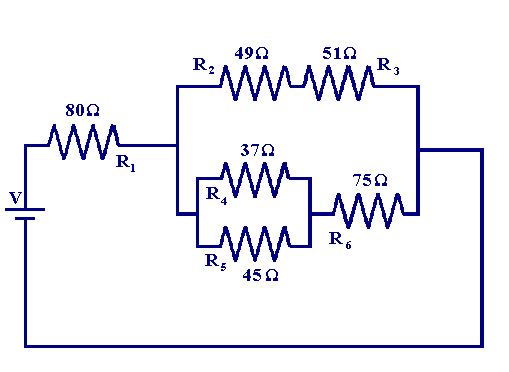What are resistor combinations?

May 28, 2014

Resistor combinations combine series and parallel paths together in a single circuit.This is a fairly simple combination circuit. To solve any combination circuit, simplify it down to a single series circuit. This is usually done most easily starting at the farthest point from the power source.

On this circuit, find the equivalent resistance of ${R}_{2}$ and ${R}_{3}$, as if they were a single resistor connected to the others in series.

$\frac{1}{R} _ T = \frac{1}{R} _ 2 + \frac{1}{R} _ 3$
$\frac{1}{R} _ T = \frac{1}{30} + \frac{1}{50}$
$\frac{1}{R} _ T = \frac{8}{150}$
Take the reciprocal of each to get ${R}_{T}$ out of the denominator:
${R}_{T} = \frac{150}{8}$
${R}_{T} = 18.75 \Omega$

Now add this to the $20 \Omega$ of ${R}_{1}$ and the $20 \Omega$ of ${R}_{2}$ to get a total resistance on this circuit of $58.75 \Omega$.

Other circuits may be more complex, and you may have to do this several times - going back and forth between series and parallel - until this is simplified down to a simple series or parallel circuit.